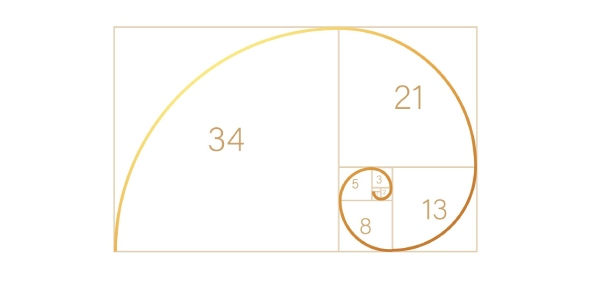# Section 3.1 – Relations

15 Questions | Attempts: 324Settings• 1.
Name the quadrant where the x-coordiante is negative and the y-coordinate is positive.
• A.

• B.

• C.

• D.

• E.

None of the above

• 2.
Joe works for Future Shop and fixes TV's.  He charges a \$50 base fee and then \$30 for each hour worked.  What would be the dependent variable for this situation?
• A.

\$50

• B.

\$30 / hour

• C.

Cost

• D.

Hours

• E.

None of the above

• 3.
The ___________________ axis can be also called the x-axis.
• 4.
Raplh works as a painter and charges \$30 for an estimate and \$20 / hour for his labour.  If someone hires him for 5 hours, how much does he roughly charge?
• A.

\$140

• B.

\$135

• C.

\$130

• D.

\$125

• E.

None of the above

• 5.
When the graph of a relation is a straight line, the relationship is called __________
• 6.
Susie works at a clothing store and earns a base salray of \$100 per week.  She also makes \$2.00 commision for every article of clothing she sells.  What would the equation be to represent her weekly pay if "E" represents her earnings per week and "c" represents every artical of clothing she sells?
• A.

E = 2c + 100

• B.

E = 100c + 2

• C.

E = 100 + 0.2c

• D.

E = 2 + 100c

• E.

None of the above

• 7.
Which graph represents the relation shown in the above table?
• A.

Top - Left

• B.

Top - Right

• C.

Bottom - Left

• D.

Bottom - Right

• 8.
Which equation represents the relation shown on the graph?
• A.

Y = -2x + 4

• B.

Y = 2x + 4

• C.

Y = x + 4

• D.

Y = -x + 4

• 9.
Which equation represents the relation shown on the graph?
• A.

Y = -2x + 18

• B.

Y = 2x + 18

• C.

Y = -4x + 18

• D.

Y = 4x + 18

• 10.
Which equation represents the relation shown in the table of values?
• A.

Y = -4x - 1

• B.

Y = 4x + 1

• C.

Y = x + 1

• D.

Y = x + 4

• 11.
Which equation represents the relation shown in the table of values?
• A.

Y = x + 10

• B.

Y = -3x - 4

• C.

Y = 3x + 4

• D.

Y = -3x + 4

• 12.
Which of these ordered pairs are points on the graph of y = 3x - 6
• A.

(2, 1)

• B.

(-3, 3)

• C.

(-1, -3)

• D.

(6, 12)

• 13.
Which of these ordered pairs are points on the graph of y = -5x + 2
• A.

(1, 3)

• B.

(-1, 7)

• C.

(-2, -8)

• D.

(4, 22)

• 14.
The graph above shows the relationship between the side length of a square and the perimeter of the square.  Use interpolation to determine the area of a square with a side length 8cm,  Which choice represents the area? (let the x-axis represent the side length and the y-axis represent the area)
• A.

25 cm^2

• B.

49 cm^2

• C.

64 cm^2

• D.

81 cm^2

• 15.
The graph above shows the relationship between the earnings Jay received and the hours be worked per week.  Use interpolation to determine the earnings Jay received for working 20 hours.  Which choice represents the earnings? (let the x-axis represent hours worked and the y-axis represent the his earnings)
• A.

\$175

• B.

\$190

• C.

\$200

• D.

\$225Back to top
×

Wait!
Here's an interesting quiz for you.